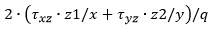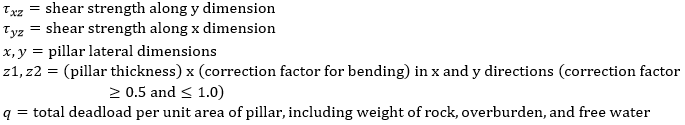RSWebinar: Designing Cost-Effective Ground Improvement Solutions with Settle3 | June 7th, 2023 Register Now

# Shear Factor of Safety

For an Elastic analysis, the Factor Safety of the rectangular pillar against vertical downward sliding is given by the ratio of the sum of the shear forces acting on the four sides of the pillar, to the total weight of the pillar, including overburden and free water, as follows:

Shear Factor of Safety =where,The form of the equation is identical to that from a Rigid analysis, the only difference being that the area on which the shear stresses act is lowered if bending stresses are high. If confining stresses are very low, the Shear Factor Safety will be about half that calculated from a Rigid analysis. As confining stress is increased, the correction factor for bending approaches 1, so at high confining stress, the Shear Factor Safety calculated from either a Rigid or Elastic analysis will be the same.

## Tension

In a Probabilistic Analysis, the Tension Count indicates the number of cases where the Shear Factor Safety was corrected for bending stresses, as a fraction of the total number of cases analyzed.

• If the first number is non-zero, then the mean Shear Factor of Safety will be less than that calculated from a Rigid analysis of the same model.
• If the first number = zero, then the mean Shear Factor of Safety will be the same from either a Rigid or an Elastic analysis.